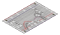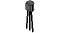﻿ products:ardx:circ-10[.:oomlout:.]

 CIRC10
 .:Temperature:..:TMP36 Precision Temperature Sensor:.(ARDX) .:Arduino Expermentation Kit:. (ARDX)

What We're Doing
What's the next phenomena we will measure with our Arduino? Temperature. To do this we'll use a rather complicated IC (integrated circuit) hidden in a package identical to our P2N2222AG transistors. It has three pin's, ground, signal and +5 volts, and is easy to use. It outputs 10 millivolts per degree centigrade on the signal pin (to allow measuring temperatures below freezing there is a 500 mV offset eg. 25 degrees C = 750 mV, 0 degrees C = 500mV). To convert this from the digital value to degrees, we will use some of the Arduino's math abilities. Then to display it we'll use one of the IDE's rather powerful features, the debug window. We'll output the value over a serial connection to display on the screen. Let's get to it.

One extra note, this circuit uses the Arduino IDE's serial monitor. To open this, first upload the program then click the button which looks like a square with an antennae.

The TMP36 Datasheet:
http://ardx.org/TMP36

The Circuit
The PartsCIRC-10Breadboard Sheet x12 Pin Headerx4TMP36Temperature Sensor x1WireSchematicResources
http://ardx.org/BBLS10

.:view:.
assembly video
http://ardx.org/VIDE10Code (no need to type everything in just)
Download the Code from ( http://ardx.org/CODE10 )
(copy the text and paste it into an empty Arduino Sketch)

/*     ---------------------------------------------------------
*     |  Arduino Experimentation Kit Example Code             |
*     |  CIRC-10 .: Temperature :. (TMP36 Temperature Sensor) |
*     ---------------------------------------------------------
*
*  A simple program to output the current temperature to the IDE's debug window
*
*  For more details on this circuit: http://tinyurl.com/c89tvd
*/

//TMP36 Pin Variables
int temperaturePin = 0; //the analog pin the TMP36's Vout (sense) pin is connected to
//the resolution is 10 mV / degree centigrade
//(500 mV offset) to make negative temperatures an option

/*
* setup() - this function runs once when you turn your Arduino on
* We initialize the serial connection with the computer
*/
void setup()
{
Serial.begin(9600);  //Start the serial connection with the copmuter
//to view the result open the serial monitor
//last button beneath the file bar (looks like a box with an antenae)
}

void loop()                     // run over and over again
{
float temperature = getVoltage(temperaturePin);  //getting the voltage reading from the tem
//perature sensor
temperature = (temperature - .5) * 100;          //converting from 10 mv per degree wit 500
// mV offset
//to degrees ((volatge - 500mV) times 100)
Serial.println(temperature);                     //printing the result
delay(1000);                                     //waiting a second
}

/*
* getVoltage() - returns the voltage on the analog input defined by
* pin
*/
float getVoltage(int pin){
return (analogRead(pin) * .004882814); //converting from a 0 to 1023 digital range
// to 0 to 5 volts (each 1 reading equals ~ 5 milliv
//olts
}

Not Working? (3 things to try)

Nothing Seems to Happen
This program has no outward indication it is working. To see the results you must open the Arduino IDE's serial monitor.
(instructions on previous page)

Gibberish is Displayed
This happens because the serial monitor is receiving data at a different speed than expected. To fix this, click the pull-down box that reads "*** baud" and change it to "9600 baud".

Temperature Value is Unchanging
Try pinching the sensor with your fingers to heat it up or pressing a bag of ice against it to cool it down.

Making it Better?

Outputting voltage:
This is a simple matter of changing one line. Our sensor outputs 10mv per degree centigrade so to get voltage we simply display the result of getVoltage().

delete the line temperature = (temperature - .5) * 100;

Outputting degrees Fahrenheit:
Again this is a simple change requiring only math. to go degrees C ----> degrees F we use the formula.
( F = C * 1.8) + 32 )
temperature = (((temperature - .5) * 100)*1.8) + 32;
before Serial.println(temperature);